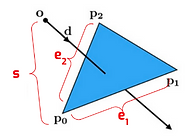top of page

# Pathtracer in Scotty3D

PathTracer is a simple path tracer that can render scenes with global illumination. The basic methodology can be divided into two parts. In the first part, we shoot a bunch of rays from the camera to the scene and check whether each ray intersects with objects. If a ray intersects the second part, we use a scattering model named bidirectional scattering distribution(BSDF) function to calculate the radiance on that intersection point.

Part 1 : Intersection detectionCamera rays are generated randomly from the camera origin, involving two space transformations. Because the generating ray function is operating in world space while it's much easier to do calculations in camera space, the transformation from world space to camera space and then back to world space is needed.Now we have the rays and are still missing the intersection detection. We need to know the locations of the intersection points. Since there are two types of primitives that the Scotty3D can take in, we need to define the intersect detection rules for both circumstances.

Up to now, the primary functions of the first part are done. However, it's inefficient and slow. That's because, for each ray, it doesn't know whether it is going to hit something in the scene or not. So, each ray has to iterate all the primitives in the scene to check whether it intersects with something. That's a tremendous amount of calculation.

To optimize, we need to introduce the bounding volume hierarchy.The bounding volume hierarchy is a binary tree structure on a set of geometric objects, which can accelerate the searching process from linear search to binary search.

The basic logic is for each model, we created a box that just right holds all the primitives. Then we divided the cube into two separate cubes according to an algorithm named Surface Algorithm Heuristic.

After recursing the process a couple of times (probably controlled by min number of primitives per box we define), all the primitives should have a parent box.

Now for each ray, we can check if the ray intersects with the root box. If not, we just ignore the ray. Else, we continuously look into the child boxes and check whether they intersect with the ray until we find the root box. Finally, we iterate all the primitives inside the root box and check whether they intersect with the ray or not.BVH demoPart 2 : Pathtracing

Now, we will simulate the complicated paths that light can take throughout the scene, bouncing off many surfaces before eventually reaching the camera. Intuitively, this is a recursive process.

To simulate this multi-bounce light, we need to use a mathematical function called bidirectional scattering distribution function(BSDF) and approximate the result by sampling using Monte Carlo integration.

For each point on the surface, there are two kinds of light we need to take into consideration. Indirect lighting is the light that bounced off at least one other surface before reaching our shading point. Direct light is the light that hits our shading point after being emitted from a light source without any bounces in between.direct light onlydirect light + indirect light

If only Lambertian material can be rendered, Scotty3D would be really boring. To add more flavour, let's add some more material to our graphics software, such as mirrors and glass. Noticing that if a material only reflects, like a perfect mirror, it is using discrete BSDF, which doesn't require Monte Carlo integration. This is because evaluating the BSDF is only necessary when sampling directions from distributions other than the BSDF itself.

For the glass material, it's a little bit more tricky. The fraction of light that is reflected vs. transmitted is governed by the dielectric (non-conductive) Fresnel equations. Instead of computing the full Fresnel equations, we can use Schlick’s approximation instead.mirror materialglass material

The last part of rendering is adding environmental lighting to the scene. An environment light is a light that supplies incident radiance from all directions on the sphere rather than using a predefined collection of explicit lights.

Firstly, we need to convert a 2D picture into a sphere using a coordinate system as the picture below.To sample the environment map, we can sample all the pixels uniformly regardless of each pixel's luminosity, which will introduce more variance. Instead, we can adopt another strategy called importance sampling, which basically gives the pixels with higher luminosity a higher chance of being sampled.uniform samplingimportance sampling

bottom of page